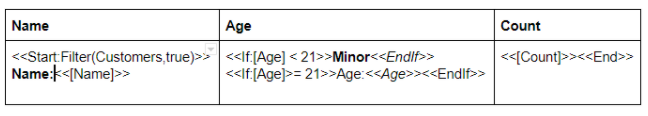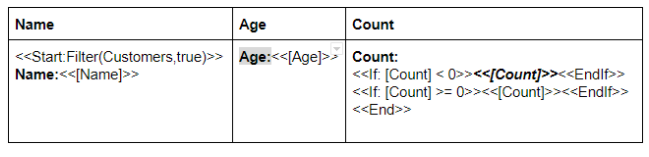# Use If expressions in templates

Use `<<If>>` expressions to conditionally include or exclude portions of a template. You can use `<<If>>` expressions in:

• Microsoft Word templates
• Email Subject, Body, and PreHeader templates
• Notify Title and Body templates
• SMS Body templates
• Webhook Body templates

Each `<<If>>` expression begins with `<<If: yesno-expression>>` and ends with `<<EndIf>>`. If the `yesno-expression` evaluates as TRUE, the template content between `<<If>>` and `<<EndIf>>` tags is included; otherwise, it is excluded.

The following sections describe ways that you can use If expressions:

## Conditionally display a field using If expressions

You can use an `<<If>>` expression to conditionally display a column value.

For example, you can display the `Address` column value if the person is at least 21 years old; otherwise, the `Address` column value is omitted:

``````<<If: ([Age] >= 21)>>
<<EndIf>>``````

Or, all in a single line:
`<<If: ([Age] >= 21)>>Address: <<[Address]>><<EndIf>>`

In the following example, the `Sales Tax` is included if `Total` is \$1.00 or more.

``````{
"Action": "Edit",
"Properties": {
"Locale": "en-US",
"Location": "47.623098, -122.330184",
"Timezone": "Pacific Standard Time"
},
"Rows": [
<<Start: Filter(OrderDetails, true)>>
{
"Name": "<<[Name]>>",
"Qty": "<<[Qty]>>",
"Price": "<<[Price]>>",
"Total": "<<[Qty]*[Price]>>",
<<If: [Qty]*[Price] >= 1.00>>
"Sales Tax": "<<[Qty]*[Price]*0.085>>",
<<EndIf>>
"Email": "<<[Email]>>",
"Product Image": "<<[Product Image]>>"
},
<<End>>
]
}``````

## Conditionally format a field using If expressions

You can use an `<<If>>` expression to conditionally format a field. For example, you can display a column's value as normal text if its value is small, or in bold text if its value is large:

``````<<If: ([Amt] < 100)>>
Amount: <<[Amt]>>
<<EndIf>>
<<If: ([Amt] >= 100)>>
Amount: <<[Amt]>>
<<EndIf>>``````

As another example, you can display a field value in red text if it is a negative value or in green text if it is positive:

``````<<If: ([Amt] < 0)>>
Amount: <<[Amt]>>
<<EndIf>>
<<If: ([Amt] >= 0)>>
Amount: <<[Amt]>>
<<EndIf>>```
```

## Display an updated field using If expressions

You can use an `<<If>>` expression to display a field when its value has changed. For example:

``````<<If: ([_THISROW_BEFORE].[Address] <> [_THISROW_AFTER].[Address])>>
<<EndIf>>```
```

## Nest If expressions

You can nest `<<If>>` expressions to arbitrary depth. For example:

``````<<If: ([Age] >= 21)>>
<<If: ([Gender] = "Male")>>
<<EndIf>>
<<EndIf>>
<<EndIf>>``````

Or, more concisely:

``````<<If: AND(([Age] >= 21), ([Gender] = "Male"), ISNOTBLANK([Address]))>>
<<EndIf>>```
```

## Include Start expressions in If expressions

You can include `<<Start>>` expressions in `<<If>>` expressions. For example:

``````<<If: COUNT([Related OrderDetails])>0>>

Order Detail Records

<<Start: [Related OrderDetails]>>

Product: <<[Product]>>
Qty: <<[Qty]>>
Amount: <<[Amount]>>

<<End>>

<<EndIf>>```
```

## Include If expressions in Start expressions

You can include `<<If>>` expressions in `<<Start>>` expressions. For example:

``````Order Detail Records

<<Start: [Related OrderDetails]>>

Product: <<[Product]>>
<<If: ([Qty] > 1)>>Qty: <<[Qty]>><<EndIf>>
Amount: <<[Amount]>>
<<End>>```
```

## Include images in If expressions

You can include images in `<<If>>` expressions. This allows you to include or exclude an image based on the value of the `<<If>>` expression. For example:

``````<<If: ISNOTBLANK([Image])>>
Image: <<[Image]>>
<<Endif>>```
```

## Include web images in If expressions

You can include web images in `<<If>>` expressions. This allows you to include or exclude an image obtained from the web based on the value of the `<<If>>` expression.

For example, when using a Google Docs template, you can conditionally include a web image as follows:

1. Open the template in Google Docs.
2. From the Insert menu, select Image, then choose an image. For example, you could select By URL to enter a URL, such as:
3. `http://cdn0.iconfinder.com/data/icons/harmonicons-02/64/check-box-512.png`
4. Click the inserted image and resize as desired.
5. Insert an `<<If>>` before the image:
6. `<<If: ([Answer] = TRUE)>>`
7. Insert an `<<Endif>>` after the image. For example:
8. `<<Endif>>`

This will include a marked checkbox image if the Answer column value is TRUE.

You can include an unmarked checkbox image if the Answer column value is FALSE, as follows:

1. Repeat the first three steps in the previous procedure, but enter the following URL:`http://chittagongit.com//images/square-icon/square-icon-13.jpg`
2. Insert an `<<If>>` before the image. For example:
`<<If: ([Answer] = FALSE)>>`
3. Insert an `<<Endif>>` after the image. For example:

`<<Endif>>`

## Include If expressions in table cells

You can include `<<If>>` expressions in table cells to include or exclude content within the cell.

The following example displays the person's `Age` if they are an adult and Minor otherwise.The following example displays the `Count` in bold italics if it negative and in normal text otherwise.